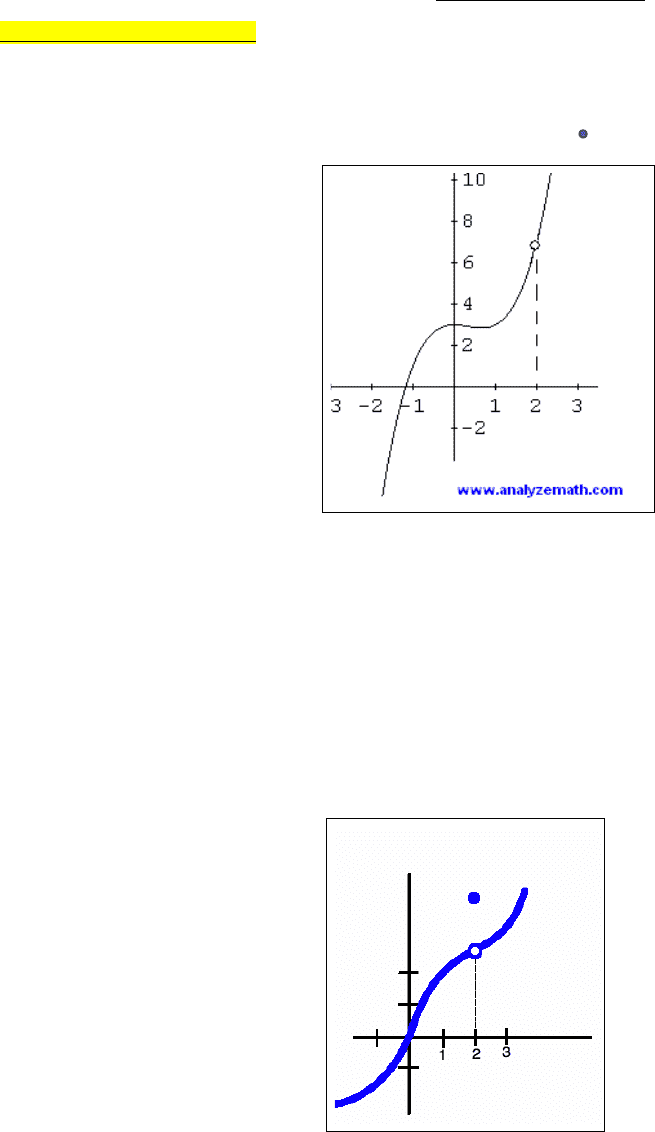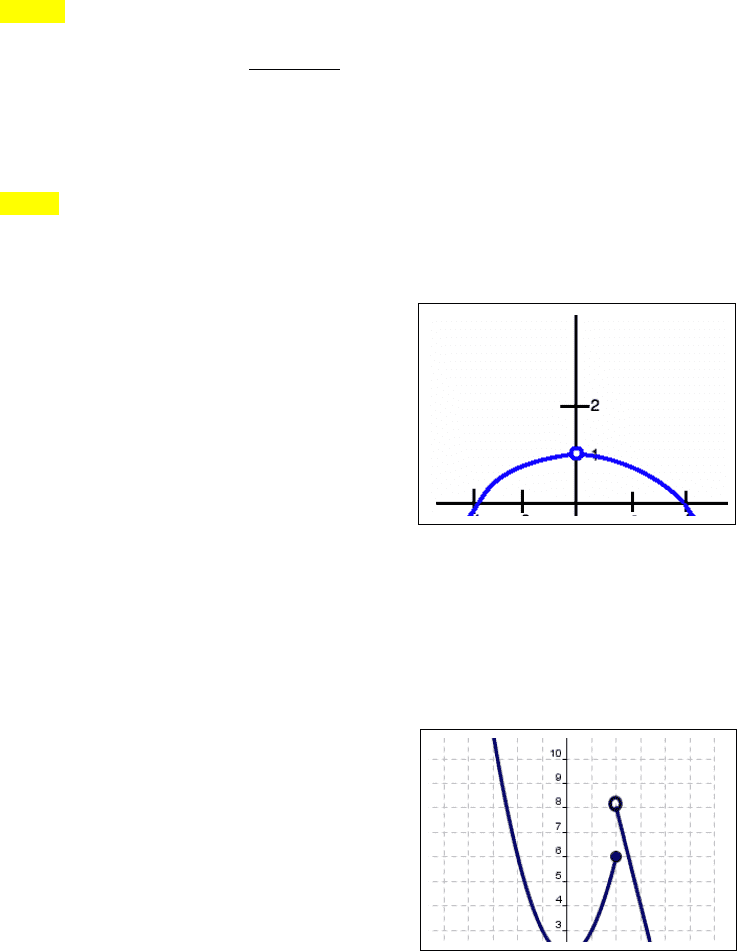Textbook Notes (280,000)
CA (170,000)
UTSC (20,000)
Chapter 10

# MATA32H3 Chapter Notes - Chapter 10: Bes, Netherlands Institute For Art History, Great News

Department
Mathematics
Course Code
MATA32H3
Professor
Raymond Grinnell
Chapter
10

This preview shows pages 1-2. to view the full 6 pages of the document.LIMITS (week 3 notes)
Topic One: Visual concept: The idea of a limit is us trying to figure out what the graph looks like, what point it hits as it
approaches the left and the right. If the graph approaches the same y-value from the left as it does from the right, then the
limit exists. However, if the graph approaches a different y-value from the left compared to the right, then the limit does
not exist (we write DNE for short).
Ex1:
In this example above, we could be asked to find:
lim
x2
f(x)
; this math language means, “please find the limit (or y-
value) of this function f(x) as x approaches 2”. You must check the graph from the left and the right to see if it approaches
the same value in order for the limit to exist. If I look from the left – that is, as x moves from the left (lets say negative 1
ish) towards the point of interest of x being 2, we see it’s hitting a y-value of 7. If I look from the right – that is, as x moves
from the right (let’s say looking from x is 3) towards the point of interest of x being 2, we see again we’re hitting a y-value
of 7. Since we approach the y-value 7 from both the left and the right then we can securely say;
lim
x2
f(x)=7
Ex2:
Here too, the limit exists despite that solid blue dot. Because we have a the graph
clearly approaching a y – value (let’s guess that it’s about y = 3 since this graph is kind of crappy) from the left and right, it
doesn’t matter that it’s an open dot (remember, open dot means we can’t touch that specific value of y = 3), we are still
approaching that y –value of 3. Therefore I can clearly state that the:
lim
x2
f(x)=3

Only pages 1-2 are available for preview. Some parts have been intentionally blurred.Note 1: The limit is different from finding
f
(
2
)
.
f
(
2
)
means that whether it’s a closed or open
dot MATTERS. It must be a closed dot for it to be the true answer. The way to find
f
(
2
)
is to recognize that the 2
represents the x-value and we want the y-value when x = 2. Because we have an open dot and closed dot at x = 2, in this
case we take the closed dot, which is a y-value of 4 let’s say (crappy graph..). So this time we say:
f
(
2
)
=4
Note 2: Based on the previous note, if we go back to the first graph where we said:
lim
x2
f(x)=7
, we could also be
f
(
2
)
.
Since there is no closed dot or solid line through x is 2, then we say
(does not exist).
Ex3:
In this example, we clearly have a limit. We can say that :
lim
x0
f(x)=1
since as x approaches zero (again
approaching and touching x are different ideas) from the left and from the
right, we approach the y-value of 1. We also can claim
f
(
0
)
=1
, since the
graph at that exact point actually has a value of y = 1.
Ex4:
In this example, we might be asked for
lim
x2
f(x).
In this case, the limit does not exist since from the left we
approach a y-value of 6, but from the right we approach a y-value of 8. However, if we are asked for
f
(
2
)
,
we can say
f
(
2
)
=6
since we have a closed dot, or exact y-value, at the moment x is 2.